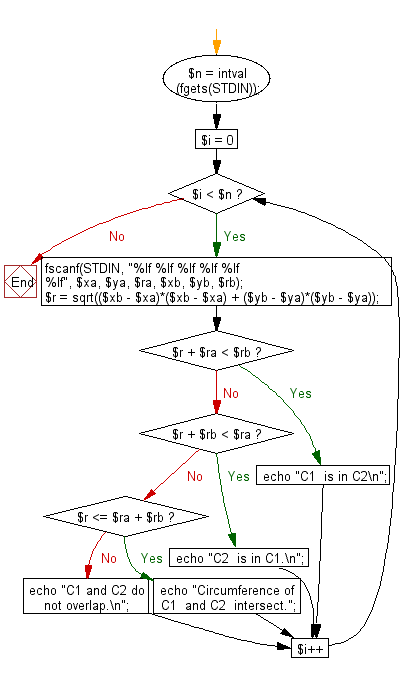﻿ PHP Exercise: Test if circumference of two circles intersect or overlap - w3resource

# PHP Exercises: Test if circumference of two circles intersect or overlap

## PHP: Exercise-58 with Solution

There are two circles C1 with radius r1, central coordinate (x1, y1) and C2 with radius r2 and central coordinate (x2, y2)
Write a PHP program to test the followings -
"C2 is in C1" if C2 is in C1
"C1 is in C2" if C1 is in C2
"Circumference of C1 and C2 intersect" if circumference of C1 and C2 intersect, and and "C1 and C2 do not overlap" if C1 and C2 do not overlap.

Input:Input numbers (real numbers) are separated by a space.
Input 0 to exit.

Sample Solution: -

PHP Code:

``````<?php
\$n = intval(fgets(STDIN));
for(\$i = 0; \$i < \$n; \$i++){
fscanf(STDIN, "%lf %lf %lf %lf %lf %lf", \$xa, \$ya, \$ra, \$xb, \$yb, \$rb);

\$r = sqrt((\$xb - \$xa)*(\$xb - \$xa) + (\$yb - \$ya)*(\$yb - \$ya));
if(\$r + \$ra < \$rb){
echo "C1  is in C2\n";
continue;
}
if(\$r + \$rb < \$ra){
echo "C2  is in C1.\n";
continue;
}

if(\$r <= \$ra + \$rb){
echo "Circumference of C1  and C2  intersect.";
continue;
}
echo "C1 and C2 do not overlap.\n";
}
?>
```
```

Sample Input:
2
0.0 0.0 6.0 0.0 0.0 5.0
0.0 0.0 3.0 5.1 0.0 3.0

Sample Output:

```C2  is in C1.
Circumference of C1  and C2  intersect.
```

Flowchart:PHP Code Editor:

Have another way to solve this solution? Contribute your code (and comments) through Disqus.

What is the difficulty level of this exercise?

Test your Programming skills with w3resource's quiz.

﻿

## PHP: Tips of the Day

PHP: How to generate a random, unique, alphanumeric string for use in a secret link?

Security Notice: This solution should not be used in situations where the quality of your randomness can affect the security of an application. In particular, rand() and uniqid() are not cryptographically secure random number generators. See Scott's answer for a secure alternative.

If you do not need it to be absolutely unique over time:

`md5(uniqid(rand(), true))`

`md5(uniqid(\$your_user_login, true))`